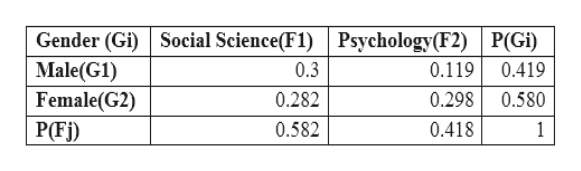# The following joint probability distribution summarize the doctoral degrees conferred in the social science and psychology by gender during one year.                                     Field                       Social Science                         Psychology                                                F1                                   F2                            P(Gi)GenderMale.           0.300                                 0.119                         0.419G1Female       0.282                                   0.298                         0.581G2P(Fj)           0.583                                   0.418                        1.000I need a. Find P (G), P(F1), and P(G1 & F1)b. Use the special multiplicacion rule to determine whether events G1 and F1 are independent

Question
29 views

The following joint probability distribution summarize the doctoral degrees conferred in the social science and psychology by gender during one year.

Field

Social Science                         Psychology

F1                                   F2                            P(Gi)

Gender

Male.           0.300                                 0.119                         0.419

G1

Female       0.282                                   0.298                         0.581

G2

P(Fj)           0.583                                   0.418                        1.000

I need

a. Find P (G), P(F1), and P(G1 & F1)

b. Use the special multiplicacion rule to determine whether events G1 and F1 are independent

check_circle

Step 1

In this question, a table has given to us which shows the joint probability distribution, based on which we have to find the probabilities.help_outlineImage TranscriptioncloseSocial Science(F1) Psychology(F2) P(Gi) Gender (Gi) Male(G1) Female(G2) 0.3 0.119 0.419 0.282 0.580 0.298 P(Fj) 0.582 0.418 1 fullscreen
Step 2

Part 1)

P(G1) = Probability of selecting a male

In the table, there is a column named P(Gi) which shows the probability of selecting male, female. Therefore, the intersection cell of P(Gi) and Male(G1) shows the probability of selecting the male = 0.419.

P(Gi) = 0.419

And P(G) = 1, because which means either selecting Male or female, the sum of probability of selecting Male or female is 1, 0.419 + 0.580 = 1.

Similarly, P(F1), where F1 denotes Social Science, probability of selecting a person with doctoral degree in Soc...

### Want to see the full answer?

See Solution

#### Want to see this answer and more?

Solutions are written by subject experts who are available 24/7. Questions are typically answered within 1 hour.*

See Solution
*Response times may vary by subject and question.
Tagged in

### Other